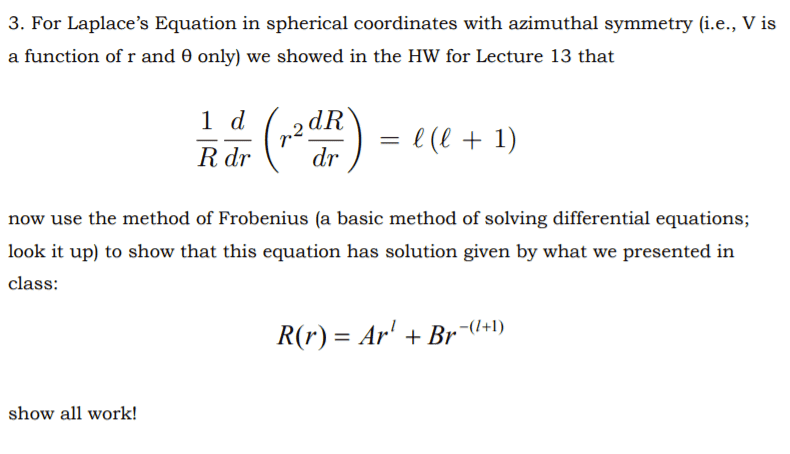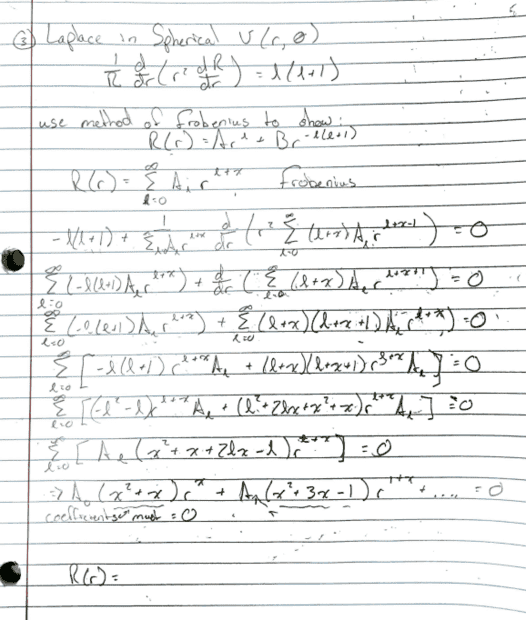# Using the Frobenius method on a 2D Laplace

jkthejetplane
Homework Statement:
I have some supplemental hmwk that I need to use a method I am not familiar with and I am not sure I have applied it correctly bc if i solve for the missing variable i will not achieve the right answer.
Relevant Equations:
Frobenius method of solving ode#### Attachments

• 2021-09-18 01-33.pdf
809.1 KB · Views: 49

Staff Emeritus
Homework Helper
The problem is you're using the symbol ##\ell## in two different ways: (1) as part of the constant that appears on the righthand side of the differential equation and (2) as the index of the summation.

•docnet
Gold Member
2022 Award
Hint:
$$\frac{\mathrm{d}}{\mathrm{d} r} \left (r^2 \frac{\mathrm{d} R}{\mathrm{d} r} \right)=r \frac{\mathrm{d}^2}{\mathrm{d} r^2} (r R)$$
helps to make the equation simpler.

Then you have to choose another summation variable in your Frobenius ansatz than ##l##, e.g.,
$$R(r)=\sum_{j=0}^{\infty} a_j r^{j+\lambda},$$
and you can assume ##a_0 \neq 0## for uniqueness. Then plug this ansatz into your ODE and determine first ##\lambda## and then the ##a_j## by comparing the coefficients in the generalized power series on both sides of your equation.

•sysprog and Delta2
jkthejetplane
Hint:
$$\frac{\mathrm{d}}{\mathrm{d} r} \left (r^2 \frac{\mathrm{d} R}{\mathrm{d} r} \right)=r \frac{\mathrm{d}^2}{\mathrm{d} r^2} (r R)$$
helps to make the equation simpler.

Then you have to choose another summation variable in your Frobenius ansatz than ##l##, e.g.,
$$R(r)=\sum_{j=0}^{\infty} a_j r^{j+\lambda},$$
and you can assume ##a_0 \neq 0## for uniqueness. Then plug this ansatz into your ODE and determine first ##\lambda## and then the ##a_j## by comparing the coefficients in the generalized power series on both sides of your equation.
Ok so as far as your hint goes, I should be using that whole expression by getting it equal to zero so I have a y" term or that I should only use the right side equal to 0?

jkthejetplane
The problem is you're using the symbol ##\ell## in two different ways: (1) as part of the constant that appears on the righthand side of the differential equation and (2) as the index of the summation.
Oh yeah i see that now. I got caught up in our notation and other notation when looking up the method. I'll try again using the standard n

Gold Member
2022 Award
Ok so as far as your hint goes, I should be using that whole expression by getting it equal to zero so I have a y" term or that I should only use the right side equal to 0?
Just plug in the ansatz into the equation and compare the coefficients of the series. You'll get an equation for ##\lambda## and then a recursion relation for the ##a_j##.

•sysprog
jkthejetplane
Update: I got it all correct after just trying again without the confusion of l terms. Did it a couple ways to make sure. Thank you

•vanhees71